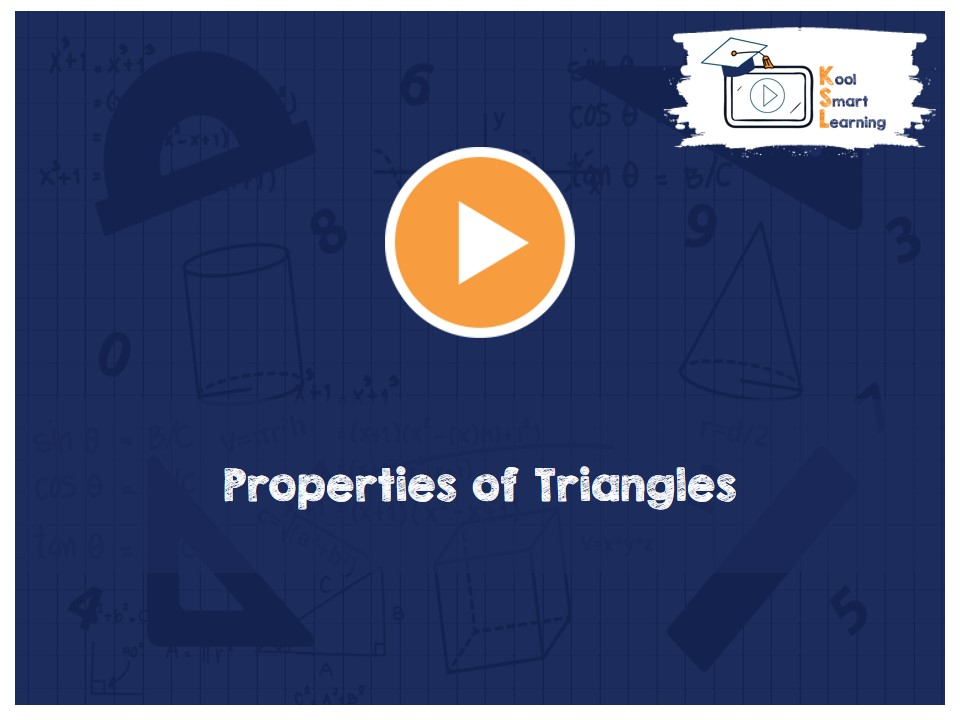At KoolSmartLearning, we intend to harness the power of online education to make learning easy.## Properties of Triangles

/  Properties of Triangles## Properties of Triangles

This video talks about the theorems related to the different properties of triangles. The proof of each theorem is also explained. The properties explained in this video are – Angles opposite to equal sides of an isosceles triangle are equal, Sides opposite to equal angles of a triangle are equal, If all sides of a triangle are equal then all its angles are also equal and If all angles of a triangle are equal then all its sides are also equal.

More Related Videos